# Effect of the Propeller on the Aircraft

## Abbreviations and Symbols

 D diameter m N yawing moment m R radius m a length of suspension lines m e half distance between suspension lines m T period of one oscillation s m mass kg g gravity acceleration m/s² n speed of rotation 1/s W rotation rate rad/s w pitch rate rad/s

## Introduction

A spinning propeller produces not only thrust but also other forces and moments. Some of these effects will be discussed here.

One main effect unique to the mechanics of rotating objects are gyroscopic moments. These moments occur when a spinning object (e.g. a metal disk, a bicycle wheel or a propeller) is more or less quickly tilted.

Rotating the front wheel of y bicycle by moving the handlebars to the "right" (right hand handle back, left handle forward) creates a "rolling moment" to the left(!). Therefore when riding a bike, a turn to the right must be initiated by briefly steering to the left - the resulting gyroscopic moment makes the bike frame lean o the right and a new force equilibrium can be achieved with the handlebar close to the neutral position again.

## Determination of the Moment of Inertia

Here we consider a typical propeller for a pylon racing model which has the following parameters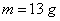,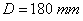,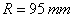in standard SI units this is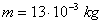,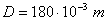,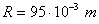The moment of inertia of the propeller can be estimated in different ways. The following paragraphs show the results of three different models.

### Modeling by Point Masses

The most simple model replaces each blade by a mass point located at the center of gravity of each blade. The center of gravity of the blades of a specific propeller was found by weighing a propeller cut in two pieces. The result was a center of gravity position at 30% of the radius. Then the moment of inertia can be determined as follows: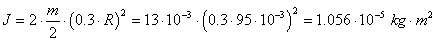The results will be a lower bound of the true value because the outer regions of the blades are not modeled with sufficient accuracy.

### Modeling by a Rod

The propeller can also be replaced by a solid rod of equal mass. Then the moment of inertia will beThis value will represent an upper limit because the blade mass is not distributed evenly along the radius - a propeller has more mass located close to the hub and less towards the tips).

### Modeling by a Disc

Finally the propeller can be thought of being replace by a solid thin disk of equal mass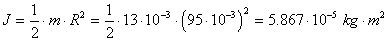Here it is assumed that the propeller mass is distributed evenly over the disc area.

### Determination by a Pendulum Experiment

The moment of inertia can also be determined by experiment. For this the propeller is suspended from two long lines and put into oscillations. From the measured period of the oscillation T for small amplitudes w can find the moment of inertia J using the following equation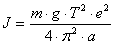A small error will be introduced by the resistance of the air, especially if the mass of the propeller is very small.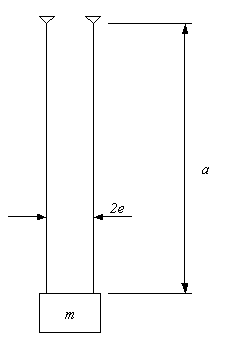Pendulum Experiment.

Using the pylon propeller (before it was cut into blades) the following values have been measured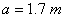,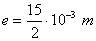,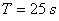These numbers produce the moment of inertia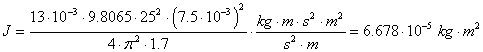While a high accuracy can be achieved with large full scale propellers, we must assume a reduced accuracy of maybe about ±20% for small lightweight model propellers.

#### Conclusions

The table shows the results of the above estimations. Except for the simple point mass model the results are fairly close. The experimental results fits between the rod and the disc model so that for practical application it seems to be justified to take the mean value of these two models.

model estimated moment of inertia
point mass J = 1.056 E-5 kg m2
rod J = 7.822 E-5 kg m2
disc J = 5.867 E-5 kg m2
experiment J = 6.678 E-5 kg m2

Comparison of different methods to estimate the moment of inertia.

In order to simplify the following calculations, a value of  J = 5.0 E-5 kg m2 has been used.

## Estimation of Yawing Moments due to Pitch Rate

The yawing moment produced by propeller precession is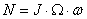Example

 propeller RPM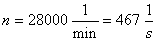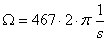moment of inertia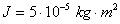pitch rate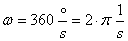(this is a violent pitch/turn maneuver, smooth coordinated turns may exhibit about half that value)

The resulting yawing moment is then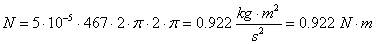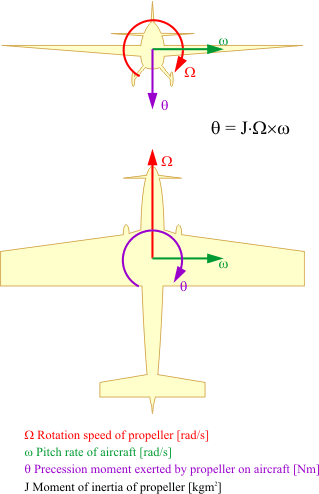In case of the common propeller sense of rotation (model aircraft: clockwise when seen from the receiver) the a "pitch up" motion w ("pull") produces a yawing moment q in the direction "rudder to the right". This moment causes the aircraft to deviate towards the right. If the pitching motion w is in the "pitch down" direction ("push") the aircraft feels a tendency to turn to the left.

If for example a the model is in in knife's edge flight orientation with the left wing down and the pilot quickly deflects the elevator "up" the model yaws to the right, turning its nose skyways.

## Torque Effect due to the Engine

Example

 propeller RPMpower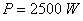The resulting torque moment at the engine shaft is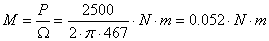This torque must be supported by the aircraft, e.g. by aileron trim. As long as the aircraft is rolling on the ground, the support is through the landing gear on the ground. Depending on track width, the wheels are loaded resp. unloaded accordingly. The different loads on the wheels produce different friction forces, and therefore a yawing moment. Assuming a track width of 200 mm the loads are uneven by +0.52 N resp. –0.52 N for the two wheels of a typical F3D model. This is a relatively small difference compared to the basic load by the model weight of about 2.5 kg (25N).

Assuming further a grass field with a friction coefficient of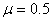the yawing moment resulting from engine torque is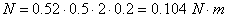This moment is only about 10% of the moment caused by rapid pitch changes as they occur at the end of the takeoff run.

## Swirl in the Propeller Wake

The wake of a propeller is swirling because of the torque of the engine. The swirling motion can be defined by the deviation of the flow direction in the wake from the flow direction outside of the wake. This swirl angle is large when the flight speed is low and becomes smaller when the flight speed is increased.

The swirling propeller wake meets the horizontal tailplanes, resulting in a rolling moment, It also interacts with the vertical fin, which is usually above the center line. This causes a side force on the fin and hence a yawing moment which must be compensated by rudder trim. Especially high powered aircraft with low RPM (high torque) produce large swirl angles at low takeoff speeds. Examples are fighters of the 1940s. Some of these aircraft therefore were equipped with vertical fins having cambered airfoil sections to avoid a constant rudder deflection for trim.

The engines of model aircraft usually run at quite high speeds which means small torque reaction moments. Therefore the swirl angle inside the wake of pylon racing aircraft is very small (less than 1 degree) and can be almost neglected.

## More Aerodynamic Effects

There are even more effects to consider in some cases. An inclined propeller at angle of attack also produces a yawing moment as well as a lift force.

The yawing moment results from the fact that the blades moving up- and down see the incoming flow at different angles - the blade on the side moving downwards (the right side on a typical model aircraft) sees a larger angle of attack and is therefore producing more thrust than when the blade is moving up on the opposite side. The average thrust of the whole propeller is hence acting with an offset to the axis of rotation. When the blades are in the vertical position, this offset is vanishing. The thrust as well as the resulting yawing moment oscillates with a frequency depending on the number of blades.

The lift force can be explained by considering the flow through the propeller disk when the propeller is at angle of attack. The streamtube passing through the propeller is bent resulting in a radial force component seen as a side force or lift.Due to the increasing amount of SPAM mail, I have to change this e-Mail address regularly. You will always find the latest version in the footer of all my pages.

It might take some time until you receive an answer and in some cases you may even receive no answer at all. I apologize for this, but my spare time is limited. If you have not lost patience, you might want to send me a copy of your e-mail after a month or so.
This is a privately owned, non-profit page of purely educational purpose. Any statements may be incorrect and unsuitable for practical usage. I cannot take any responsibility for actions you perform based on data, assumptions, calculations etc. taken from this web page.